# Regular Graph in Graph Theory

Prerequisite: Graph Theory Basics – Set 1, Set 2

Regular Graph:
A graph is called regular graph if degree of each vertex is equal. A graph is called K regular if degree of each vertex in the graph is K.

Example:
Consider the graph below: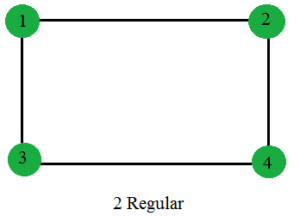Degree of each vertices of this graph is 2. So, the graph is 2 Regular. Similarly, below graphs are 3 Regular and 4 Regular respectively.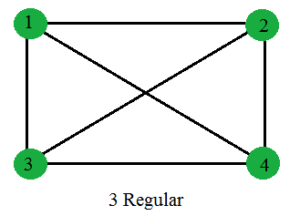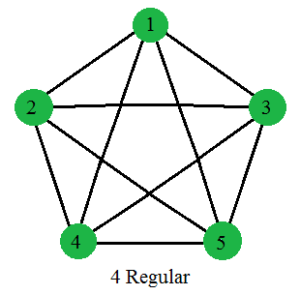Properties of Regular Graphs:

1. A complete graph N vertices is (N-1) regular.
Proof:
In a complete graph of N vertices, each vertex is connected to all (N-1) remaining vertices. So, degree of each vertex is (N-1). So the graph is (N-1) Regular.

2. For a K Regular graph, if K is odd, then the number of vertices of the graph must be even.
Proof:
Lets assume, number of vertices, N is odd.
From Handshaking Theorem we know,
Sum of degree of all the vertices = 2 * Number of edges of the graph …….(1)
The R.H.S of the equation (1) is a even number.

For a K regular graph, each vertex is of degree K. Sum of degree of all the vertices = K * N, where K and N both are odd.So their product (sum of degree of all the vertices) must be odd. This makes L.H.S of the equation (1) is a odd number. So L.H.S not equals R.H.S. So our initial assumption that N is odd, was wrong. So, number of vertices(N) must be even.

3. Cycle(Cn) is always 2 Regular.
Proof:
In Cycle (Cn) each vertex has two neighbors. So, they are 2 Regular.

4. 2 Regular graphs consists of Disjoint union of cycles and Infinite Chains.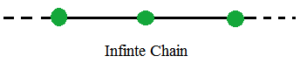5. Number of edges of a K Regular graph with N vertices = (N*K)/2.
Proof:
Let, the number of edges of a K Regular graph with N vertices be E.
From Handshaking Theorem we know,

Sum of degree of all the vertices = 2 * E
N * K = 2 * E
or, E = (N*K)/2

6. A K-dimensional Hyper cube (Qk) is a K Regular graph.
Below is a 3-dimensional Hyper cube(Q3) which is a 3 Regular graph.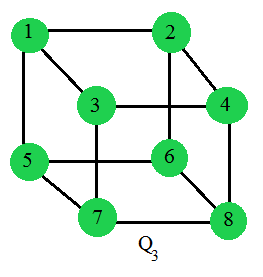My Personal Notes arrow_drop_upCheck out this Author's contributed articles.

If you like GeeksforGeeks and would like to contribute, you can also write an article using contribute.geeksforgeeks.org or mail your article to contribute@geeksforgeeks.org. See your article appearing on the GeeksforGeeks main page and help other Geeks.

Please Improve this article if you find anything incorrect by clicking on the "Improve Article" button below.

Article Tags :

Be the First to upvote.

Please write to us at contribute@geeksforgeeks.org to report any issue with the above content.